Get instant live expert help with Excel or Google Sheets“My Excelchat expert helped me in less than 20 minutes, saving me what would have been 5 hours of work!”

#### Post your problem and you’ll get expert help in seconds.

Your message must be at least 40 characters
Our professional experts are available now. Your privacy is guaranteed.

# Excel Data Validation Istext Function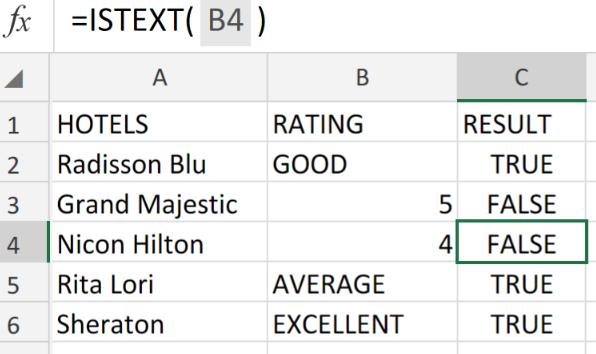Figure 1. Excel Data Validation Istext Function.

If we want to permit text entries only in the cells of our Excel sheet, we are going to utilize data validation with a custom formula derived from the ISTEXT function

## Formula Syntax

`=ISTEXT(A1)`

This formula is useful for restricting users from populating cells with numbers, dates, etc.

## How to use the Excel Data Validation Istext Function.

The ISTEXT formula will check if the value entered is a text string. If the condition is met, it return a TRUE result, and if the condition is not met, a FALSE result.

We can get this done with 3 simple steps;

1. Arrange the data available for validation within labeled columns of our worksheet.

See example illustrated below;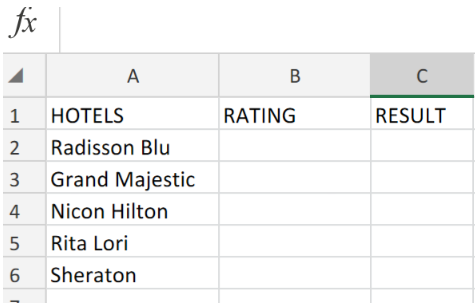Figure 2. Excel Data Validation Istext Function.

Our purpose here is to permit ratings to be entered in text format only.

Be sure to provide empty cells for Excel to generate the results.

1. In our worksheet example above, we will enter the following ISTEXT formula into cell C2;

`=ISTEXT(B2)`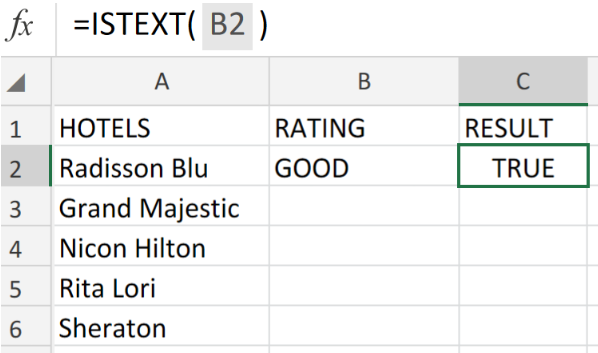Figure 3. Excel Data Validation Istext Function.

Since the data entry in cell B2 of our worksheet example, the Excel ISTEXT formula returned a TRUE result in cell C2.

1. Copy a modified version of the ISTEXT formula in cell C2, down the other cells in the RESULT column for the other results to be generated.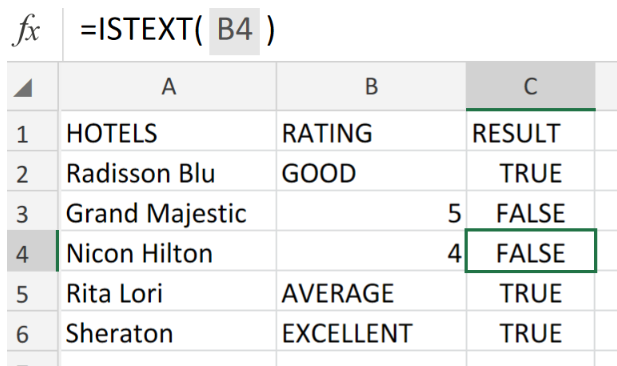Figure 4. Excel Data Validation Istext Function.

Whenever a user adds or changes a cell value, data validation rules are triggered.

This means that, all inputted text will pass validation, however, formulas and numbers will not pass validation.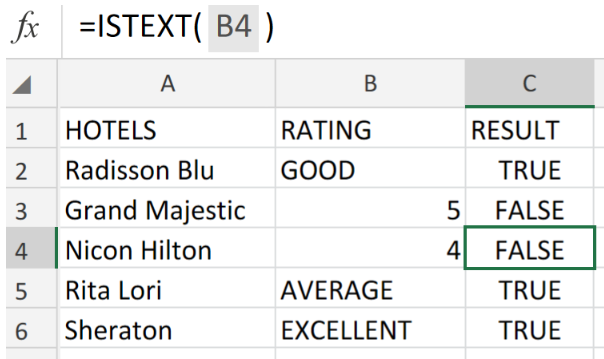Figure 5. Final Result.

## Instant Connection to an Expert through our Excelchat Service:

Our live Excelchat Service is here for you. We have Excel Experts available 24/7 to answer any Excel questions you may have. Guaranteed connection within 30 seconds and a customized solution for you within 20 minutes.

### Did this post not answer your question? Get a solution from connecting with the expert.Another blog reader asked this question today on Excelchat:
Related blogs
Solution examplesUse the Vlookup Function to complete the "employee" column of table 2. Use "job Id" from table 2 as your lookup_value(s) and table 1 as your reference.
Solved by C. H. in 16 minsIf a cell in another sheet is populated I need a vlookup done. If the cell is not populated I need the cell to return blank.
Solved by T. D. in 60 minsI am trying to make a chart that turns a week range red if nothing is entered in the range. If something is entered then I would like it to turn green. Please Help
Solved by E. U. in 43 minsI need a check box to show/hide an answer of an if function
Solved by Z. U. in 23 minsI need a formula to compare the data in two columns and then export the mismatched data in the 3rd column
Solved by S. Q. in 20 mins## Subscribe to Excelchat.coAnother blog reader asked this question today on Excelchat: